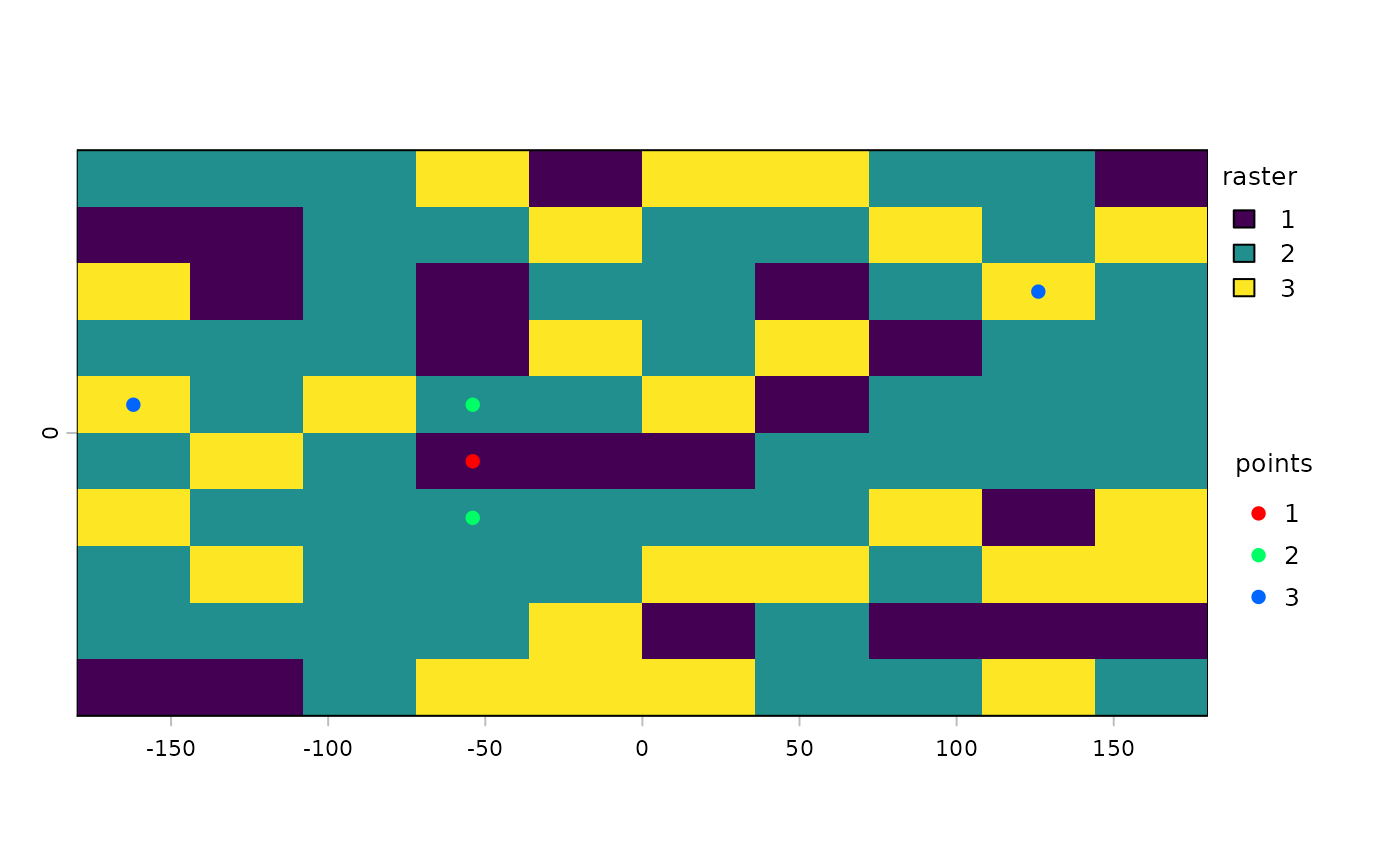Take a spatial sample from a SpatRaster, SpatVector or SpatExtent. Sampling a SpatVector or SpatExtent always returns a SpatVector of points.

With a SpatRaster, you can get cell values, cell numbers (cells=TRUE), coordinates (xy=TRUE) or (when type="regular" and as.raster=TRUE) get a new SpatRaster with the same extent, but fewer cells.

In order to assure regularity when requesting a regular sample, the number of cells or points returned may not be exactly the same as the size requested.

## Usage

# S4 method for SpatRaster
spatSample(x, size, method="random", replace=FALSE, na.rm=FALSE,
as.raster=FALSE, as.df=TRUE, as.points=FALSE, values=TRUE, cells=FALSE,
xy=FALSE, ext=NULL, warn=TRUE, weights=NULL, exp=5, exhaustive=FALSE)

# S4 method for SpatVector
spatSample(x, size, method="random", strata=NULL, chess="")

# S4 method for SpatExtent
spatSample(x, size, method="random", lonlat, as.points=FALSE)

## Arguments

x

SpatRaster, SpatVector or SpatExtent

size

numeric. The sample size. If x is a SpatVector, you can also provide a vector of the same length as x in which case sampling is done separately for each geometry. If x is a SpatRaster, and you are using method="regular" you can specify the size as two numbers (number of rows and columns)

method

character. Should be "regular" or "random", If x is a SpatRaster, it can also be "stratified" (each value in x is a stratum) or "weights" (each value in x is a probability weight)

replace

logical. If TRUE, sampling is with replacement (if method="random")

na.rm

logical. If TRUE, NAs are removed. Only used with random sampling of cell values. That is with method="random", as.raster=FALSE, cells=FALSE

as.raster

logical. If TRUE, a SpatRaster is returned

as.df

logical. If TRUE, a data.frame is returned instead of a matrix

as.points

logical. If TRUE, a SpatVector of points is returned

values

logical. If TRUE raster cell values are returned

cells

logical. If TRUE, cell numbers are returned. If method="stratified" this is always set to TRUE if xy=FALSE

xy

logical. If TRUE, cell coordinates are returned

ext

SpatExtent or NULL to restrict sampling to a subset of the area of x

warn

logical. Give a warning if the sample size returned is smaller than requested

weights

SpatRaster. Used to provide weights when method="stratified"

strata

if not NULL, stratified random sampling is done, taking size samples from each stratum. If x has polygon geometry, strata must be a field name (or index) in x. If x has point geometry, strata can be a SpatVector of polygons or a SpatRaster

chess

character. One of "", "white", or "black". For stratified sampling if strata is a SpatRaster. If not "", samples are only taken from alternate cells, organized like the "white" or "black" fields on a chessboard

lonlat

logical. If TRUE, sampling of a SpatExtent is weighted by cos(latitude). For SpatRaster and SpatVector this done based on the crs, but it is ignored if as.raster=TRUE

exp

numeric >= 1. "Expansion factor" that is multiplied with size to get an initial sample used for stratified samples and random samples with na.rm=TRUE to try to get at least size samples

exhaustive

logical. If TRUE and na.rm=TRUE first all cells that are not NA are determined and a sample is taked from these cells. This is useful when you are dealing with a very large raster that is sparse (most cells are NA). Otherwise, the default approach may not find enough samples. This should not be used in other cases, especially not with large rasters that mostly have values

## Value

numeric matrix, data.frame, SpatRaster or SpatVector

## Examples

f <- system.file("ex/elev.tif", package="terra")
r <- rast(f)
s <- spatSample(r, 10, as.raster=TRUE)
spatSample(r, 5)
#>   elevation
#> 1       267
#> 2        NA
#> 3       462
#> 4       224
#> 5       272
spatSample(r, 5, na.rm=TRUE)
#>   elevation
#> 1       492
#> 2       410
#> 3       330
#> 4       292
#> 5       291
spatSample(r, 5, "regular")
#>   elevation
#> 1       479
#> 2       NaN
#> 3       NaN
#> 4       419
#> 5       290
#> 6       306
#> 7       281
#> 8       286
#> 9       NaN

## if you require cell numbers and/or coordinates
size <- 6
spatSample(r, 6, "random", cells=TRUE, xy=TRUE, values=FALSE)
#>      cell        x        y
#> [1,] 3193 6.220833 49.91250
#> [2,]  869 5.854167 50.11250
#> [3,] 2227 6.087500 49.99583
#> [4,] 4675 5.904167 49.77917
#> [5,] 8234 6.270833 49.47083
#> [6,] 3784 6.395833 49.86250

# regular, with values
spatSample(r, 6, "regular", cells=TRUE, xy=TRUE)
#>   cell        x        y elevation
#> 1 7458 6.137500 49.53750       264
#> 2 7505 6.529167 49.53750        NA
#> 3 7411 5.745833 49.53750        NA
#> 4 5368 6.137500 49.72083       289
#> 5 5415 6.529167 49.72083        NA
#> 6 5321 5.745833 49.72083        NA
#> 7 3183 6.137500 49.91250       322
#> 8 1093 6.137500 50.09583        NA

# stratified
rr <- rast(ncol=10, nrow=10, names="stratum")
set.seed(1)
values(rr) <- round(runif(ncell(rr), 1, 3))
spatSample(rr, 2, "stratified", xy=TRUE)
#>      x   y stratum
#> 1 -126  63       1
#> 2  126 -27       1
#> 3  126  27       2
#> 4 -162  81       2
#> 5   18 -45       3
#> 6  -18  27       3

s <- spatSample(rr, 5, "stratified", as.points=TRUE)
plot(rr, plg=list(title="raster"))
plot(s, 1, add=TRUE, plg=list(x=185, y=1, title="points"))## SpatExtent
e <- ext(r)
spatSample(e, 10, "random", lonlat=TRUE)
#>              x        y
#>  [1,] 6.362453 49.60080
#>  [2,] 6.214008 50.07085
#>  [3,] 5.841767 49.53690
#>  [4,] 5.832523 50.14225
#>  [5,] 6.160047 49.56096
#>  [6,] 6.504415 49.51819
#>  [7,] 6.188561 49.58855
#>  [8,] 6.299401 49.90880
#>  [9,] 6.361697 49.46779
#> [10,] 6.250035 49.81599

## SpatVector
f <- system.file("ex/lux.shp", package="terra")
v <- vect(f)

# sample the geometries
i <- sample(v, 3)

# sample points in geometries
p <- spatSample(v, 3)